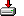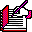#loaddata.sas```/* Richard A. DeVenezia
* January 29, 2004
*
* Very simple cards like emulation for DATA Steps emitted by macro
*/
```
```%macro loaddata (

input=        %* input statement (without the input);
, data=         %* data to read values from;
, debug=        %* any single DATA Step statement - typically a put;
, limit=1000    %* maximum number of rows to output - also prevents infinite loops;
, lrecl=5000    %* size of _infile_ buffer - should be large enough to contain data;
, bufferef=     %* the fileref used to obtain _infile_ buffer;

);

%*
%* Works on the presumption that input mates well with data
%* After inputting last block of values the _infile_ column pointer
%* will be at artifical end-of-data marker FF
%*;

infile &bufferef lrecl=&lrecl col=_column_;

input @;

_infile_ = "&data." || "20FF"X;
_infile_len_ = length (_infile_); drop _infile_len_;

_n_ = 0;
do while (_error_ = 0);
_n_ + 1;

input &input @;

&debug;

output;

if _column_ = _infile_len_ then leave;

if _n_ > &limit then stop; * prevent infinte loops;
end;

%mend;```

Sample code

```%macro foo;
filename null pipe "echo";
data a;
bufferef = null
, input= x y1 y2 y3
, debug= put x y1 y2 y3
, data=
1 1 2 3
2 3 4 5
3 4 5 6
4 5 6 7
5 6 7 8
6 7 8 9
7 8 9 10
)
run;
filename null ;
%mend;

options mprint;

%foo```